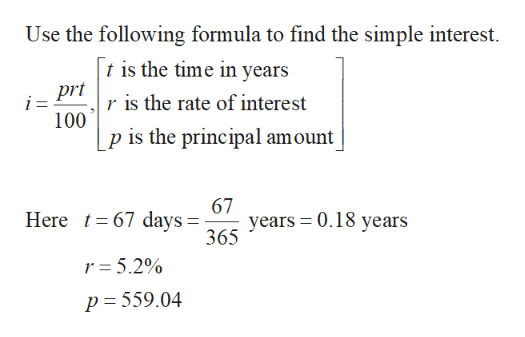# p=559.04,r = 5.2%, t= 67 daysthe simple interest rounded to the nearest cent if needed

Question
22 views

p=559.04,r = 5.2%, t= 67 days

the simple interest rounded to the nearest cent if needed

check_circle

Step 1

Obtain the simple interest for the given details as follows.

r = 5....help_outlineImage TranscriptioncloseUse the following formula to find the simple interest t is the time in years pr is the rate of interest i= 100 p is the principal amount 67 years 0.18 years 365 Here 67 days = r = 5.2% p = 559.04 fullscreen

### Want to see the full answer?

See Solution

#### Want to see this answer and more?

Solutions are written by subject experts who are available 24/7. Questions are typically answered within 1 hour.*

See Solution
*Response times may vary by subject and question.
Tagged in

### Math